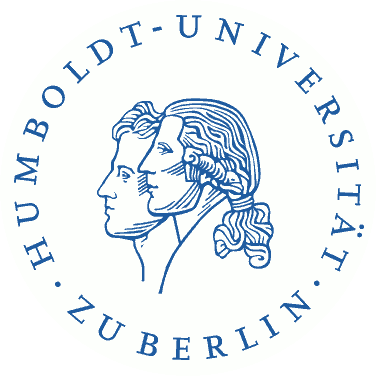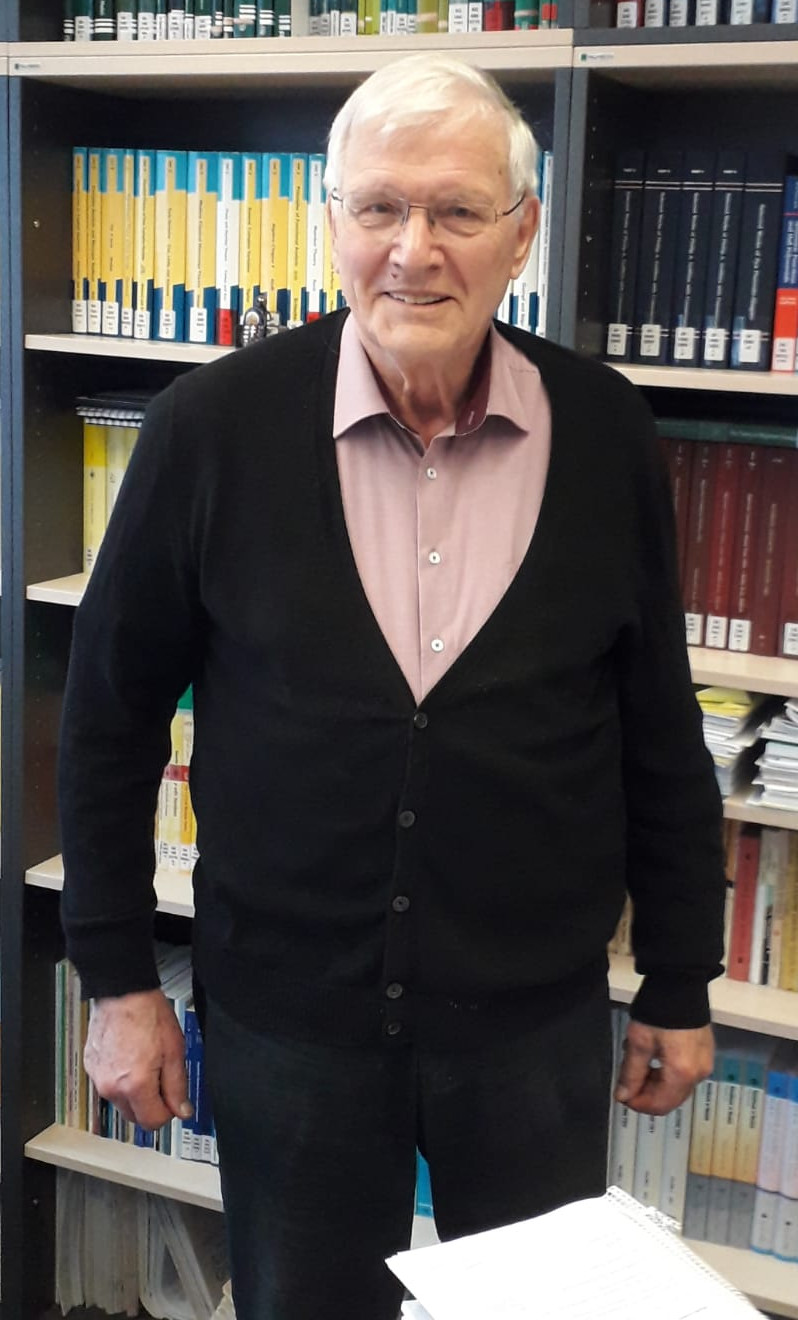Complex Algebraic Geometry# Complex Algebraic Geometry

## A meeting for Herbert Kurke's 80th birthday## Program

13:00 - 14:00: Klaus Hulek: O'Grady manifolds of dimension 10: moduli and topology
Irreducible symplectic holomorphic manifolds (aka as hyperkähler manifolds) are one of the three building blocks of Ricci flat compact Kähler manifolds. Until now one only knows two series of such manifolds, namely manfolds of $$K3^{[n]}$$-type and generalized Kummer varieties as well as two special examples, the O'Grady manifolds of dimension $$6$$ and $$10$$. In this talk I will discuss some aspects concerning the moduli and the topology of these varieties.
14:30 - 15:30: Klaus Altmann: Displaying the cohomology of toric line bundles
Line bundles $$L$$ on projective toric varieties can be understood as formal differences $$\Delta^+ - \Delta^-$$ of convex polyhedra in the character lattice. We show how it is possible to use this language for understanding the cohomology of $$L$$ by studying the set-theoretic difference $$\Delta^- \setminus \Delta^+$$.
16:00 - 17:00: Michael Rapoport: Moduli spaces of abelian varieties and their $$p$$-adic analogues
Moduli spaces of abelian varieties belong to the most studied algebraic varieties. They are also the most accessible Shimura varieties, i.e., roughly speaking, hermitian locally symmetric spaces. Since their definition by Deligne in 1971, Shimura varieties have played an important role in algebraic geometry and number theory. Now let $$p$$ be a prime number. In my talk I will address the question of defining $$p$$-adic analogues of Shimura varieties. They are rigid-analytic varieties over $$p$$-adic fields, and are expected to play a role similar to Shimura varieties in $$p$$-adic algebraic geometry and $$p$$-adic number theory. Scholze recently defined local Shimura varieties and showed that Rapoport-Zink formal moduli spaces of $$p$$-divisible groups give examples of local Shimura varieties. I will discuss this circle of ideas.

## Social Dinner

The social dinner will take place at 19:00 at the Restaurant Hasir Mitte, Oranienburger Str. 4, 10178 Berlin.

If you would like to participate, please send an email to Désirée Dzomo Quevedo at dzomoqud@math.hu-berlin.de.